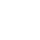## Air Blowers Calculation

4. Total pressure and total pressure coefficient of air blowers:

(1) Total pressure of blower: the difference between the total pressure on the outlet section and the total pressure on the inlet section. Expressed in PTF, commonly used unit: PA

(2) Total pressure coefficient: ψ t = kpptf / ρ u22, where ψ T: total pressure coefficient Kp: compressibility correction coefficient PTF: total pressure of blower, PA ρ: gas density at inlet of blower, kg / m ^ 3 U2: linear velocity of impeller outer edge, M / s

3. Flow and flow coefficient of air blowers

(1) Flow: refers to the volume of gas flowing through the inlet of air blowers in unit time. Expressed in Q, usually in m3 / h or m3 / min.

(2) Flow coefficient: φ = q / (900 π D22 × U2), where: φ: flow coefficient, Q: flow, m3 / h, D2: impeller diameter, mu2: linear velocity of impeller outer edge, M / S (U2 = π D2N / 60)

1、 air blowers selection basic knowledge :

(1)Standard state: refers to the air pressure at the inlet of air blowers P = 101325pa, temperature T = 20 ℃, relative humidity φ = 50%.

(2) Specified state: refers to the air intake condition of the air blowers. This includes the local atmospheric pressure or altitude, the pressure of the inlet gas, the temperature of the inlet gas, and the composition and volume percentage concentration of the inlet gas.

2、 Example of model selection (only one example)

Select an induced draft fan for 2T / h industrial boiler. The performance parameters of the blower and the corresponding air intake conditions at the maximum load are as follows:

Flow: q = 6800 m3 / h, inlet temperature: T1 = 200 ℃

Total pressure: PTF = 2010 PA, inlet absolute pressure P = 96000 PA

Solution: (1) flow rate per second: QS = 6800 / 3600 = 1.89 m3 / s

(2) Air density under specified conditions: ρ = P / RT = 96000 / (287 × (273 + 200)) = 0.707 kg / m3

(3) Converted to total pressure under standard state: ptf0 = PTF × ρ 0 / ρ = 2010 × 1.2/0.707 = 3412 PA

(4) Selected air blowers spindle speed: n = 2800 R / min

(5) Calculation of compressibility correction coefficient:

Kp=K/（K－1）[（1+PtF/P）((k－1)/k)－1]×(PtF/P)－1

=1.4/(1.4－1) ×[(1+2010/96000)(1.4－1)/1.4－1] ×(2010/96000)－1

=0.9926